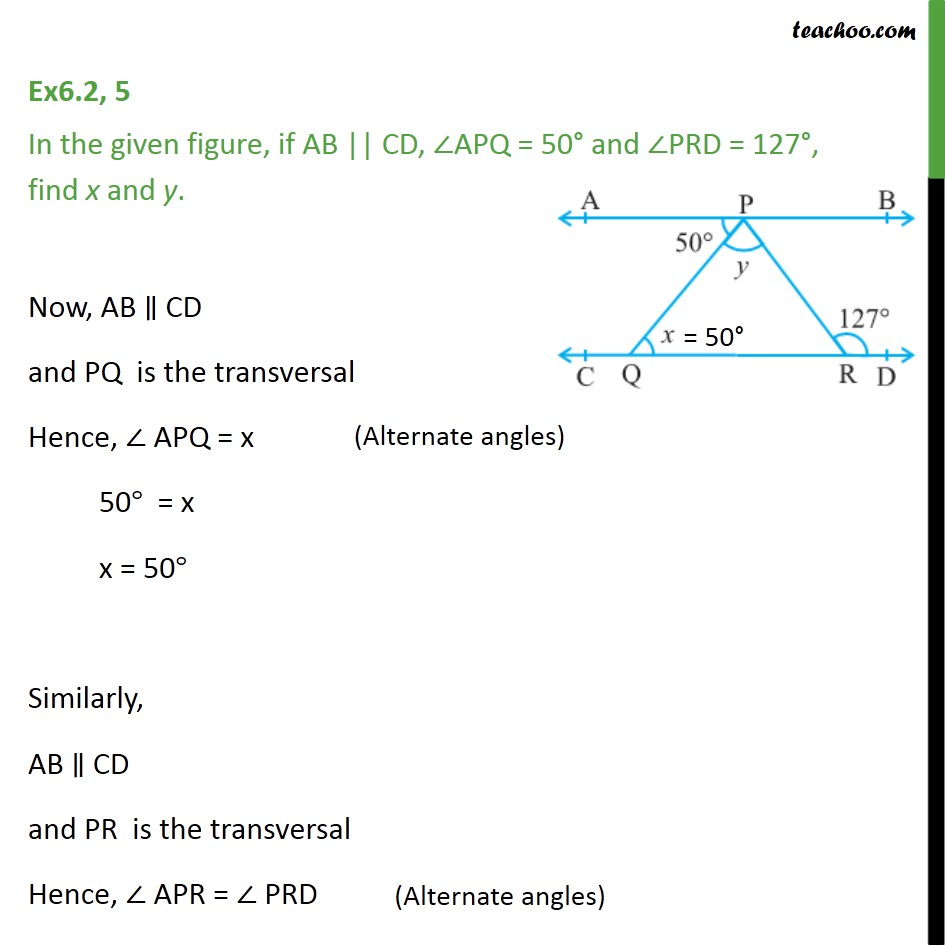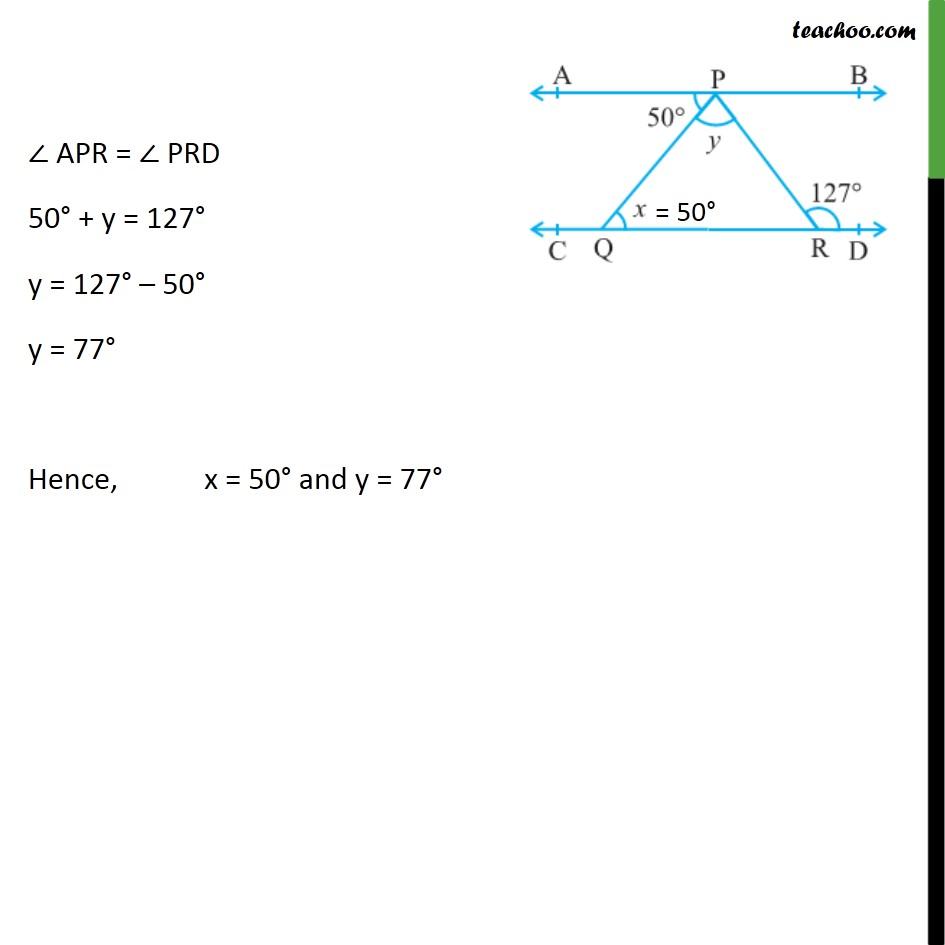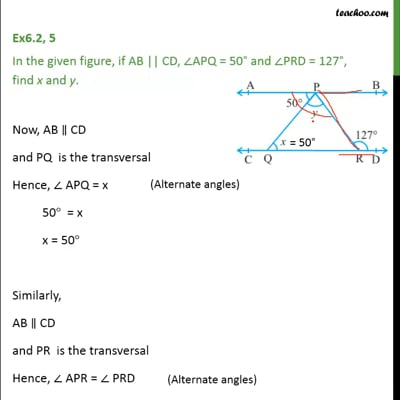Ex 6.2

Chapter 6 Class 9 Lines and Angles
Serial order wiseThis video is only available for Teachoo black users

Maths Crash Course - Live lectures + all videos + Real time Doubt solving!

### Transcript

Ex6.2, 5 In the given figure, if AB || CD, APQ = 50 and PRD = 127 , find x and y. Now, AB CD and PQ is the transversal Hence, APQ = x 50 = x x = 50 Similarly, AB CD and PR is the transversal Hence, APR = PRD APR = PRD 50 + y = 127 y = 127 50 y = 77 Hence, x = 50 and y = 77